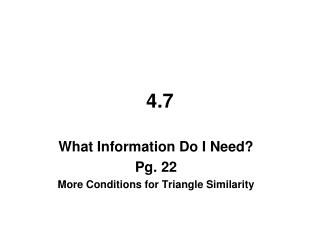# 4.7 - PowerPoint PPT PresentationDownload Presentation4.7

4.7Download Presentation## 4.7

- - - - - - - - - - - - - - - - - - - - - - - - - - - E N D - - - - - - - - - - - - - - - - - - - - - - - - - - -
##### Presentation Transcript

1. 4.7 What Information Do I Need? Pg. 22 More Conditions for Triangle Similarity

2. 4.7 – What Information Do I Need? More Conditions for Triangle Similarity So far, you have worked with two methods for determining that triangles are similar: AA~ and SSS~. Are these the only ways to determine if two triangles are similar? Today you will investigate similar triangles and complete your list of triangle similarity conjectures.

3. 4.36 – ANOTHER WAY Richard's team is using SSS~ Conjecture to show that two triangles are similar. "This is too much work," Richard says. "When we're using the AA~ Conjecture, we only need to look at two angles. Let's just calculate the ratios for two pairs of corresponding sides to determine that triangles are similar."

4. Is SS~ a valid similarity conjecture for triangles? That is, if two pairs of corresponding side lengths share a common ratio, must the triangles be similar? In this problem you will investigate this question using a manipulative or a dynamic geometry tool No SS~

5. 5 10 a. Richard has a triangle with side lengths 5cm and 10cm. If your triangle has two sides that share a common ratio with Richards, does your triangle have to be similar to his? No

6. 5 10 b. Kirk asks, "What if the angles between the two sides have the same measure? Would that be enough to know the triangles are similar?" Yes

7. c. Kirk calls this the "SAS~ Conjecture," placing the "A" between the two "S"s because the angle is between the two sides. He knows it works for Richard's triangle, but does it work on all other triangles? SAS~ Yes, SAS~

8. 4.37 – SSA~ or ASS~ Cori's team put "SSA~" on their list of possible triangle similarity conjectures. Investigate if this is a valid similarity conjecture. If a triangle has two sides sharing a common ratio with Richard's, and has the same angle "outside" these sides, must it be similar?

9. 5 10 5 10 SSA~ or ASS~ There is no ASS or SSA in geometry!

10. 4.38 – ANYTHING ELSE? What other triangle similarity conjectures involving sides and angles might there be? List the names of every other possible triangle similarity conjecture you can think of that involves sides and angles. AAA~ SSA~ SSS~ AAS~ SAS~ ASA~ ASS~ SAA~

11. 4.39 – AAS~ or SAA~ Betsy's team came up with a similarity conjecture they call "AAS~," but Betsy thinks they should cross it off their list. Betsy says, "This similarity conjecture has extra, unnecessary information There is no point in having it on our list.” a. What is Betsy talking about? Why does the AAS~ method contain more information than you need? AA~ is enough

12. b. Go through your list of possible triangle similarity conjectures, crossing off all the invalid ones and all the ones that contain unnecessary information. AAA~ SSA~ SSS~ AAS~ SAS~ ASA~ ASS~ SAA~

13. c. How many valid triangle similarity conjectures are there? List them. AAA~ SSA~ SSS~ AAS~ SAS~ ASA~ ASS~ SAA~ 3 AA~ SSS~ SAS~

14. 4.40 – FLOWCHARTS Lynn wants to show that the triangles are similar. a. What similarity conjecture should Lynn use? SAS~

15. 8 16 3 6 1 2 1 2 = = given given given ΔKLM ΔABC ~ SAS~

16. 4.41 – USING SIMILARITY Examine the triangles. a. Are these triangles similar? If so, make a flowchart justifying their similarity. Hint: It might help to draw the triangles separately first.

17. E D 27 15 36 25° C G 25° 60° 20 C F 36 15 27 5 9 20. 36 5 9 = = given given given ΔGCD ~ ΔFCE SAS~

18. E D 27 15 36 25° C G 25° 60° 20 C F 36 Both are correct!

19. c. Find all the missing side lengths and all the missing angle measures in the two triangles. E D 95° 27 15 95° x 36 60° 25° C G 25° 60° 20 C F 36 27x = 540 x = 20

20. 4.42 – FLOWCHARTS Write a flowchart to prove the following triangles are similar.

21. Y given given ΔNTM ~ ΔXYB AA~

22. 9 6 3 2 12. 8 3 2 15 10 3 2 = = = given given given ΔABC ~ ΔDEF SSS~

23. If _____ __________________angles are _________, then the triangles are similar by AA~. two corresponding equal X A B Y

24. If _________ ____________________ sides are _______________________, then the triangles are similar by SSS~. three corresponding proportional z c a x y b b y c z a x = =

25. two corresponding If _____ _____________________ sides are _________________and the angle _________________ them is ____________, then the triangles are similar by SAS~. proportional between equal x a B Y z c a x c z =